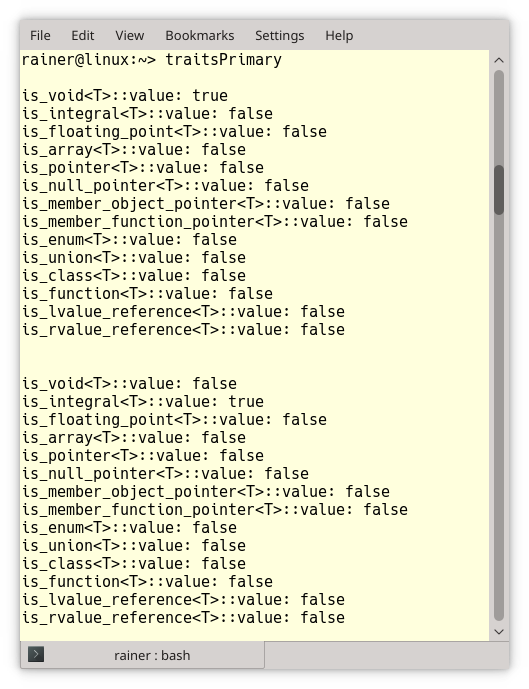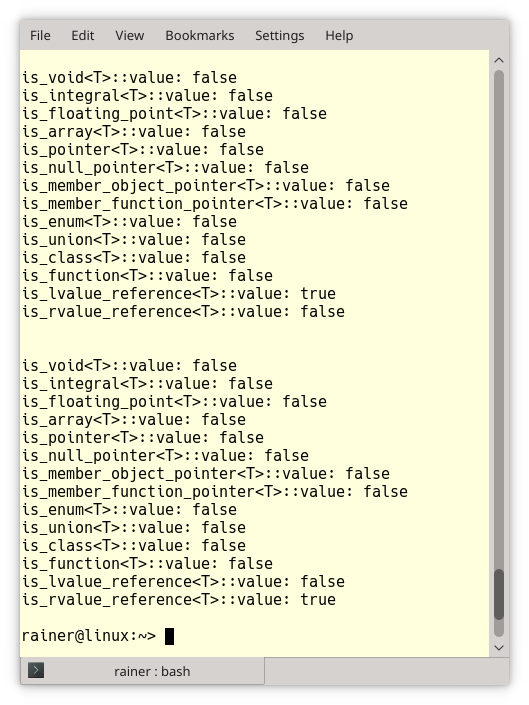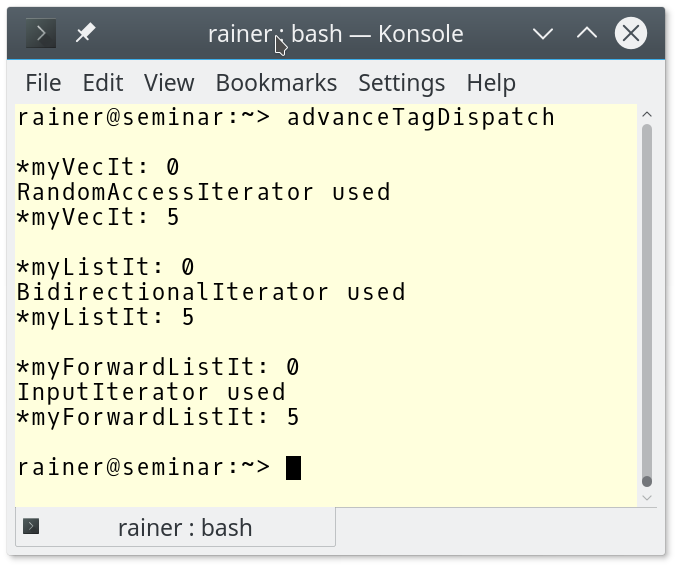# C++ Core Guidelines: Definition of Concepts, the Second

Let me continue with the rules for defining concepts in the guidelines. The first of the three remaining rules in this post is quite sophisticated.Here are the rules for today:

The explanation of the first rules is relatively concise. Maybe, too concise.

## T.24: Use tag classes or traits to differentiate concepts that differ only in semantics

This is the reason for this rule from the guidelines: “Two concepts requiring the same syntax but having different semantics leads to ambiguity unless the programmer differentiates them.”

##Modernes C++ Mentoring

Be part of my mentoring programs:

• "Fundamentals for C++ Professionals" (open)
• "Design Patterns and Architectural Patterns with C++" (open)
• "C++20: Get the Details" (reopens December 2023)
• Do you want to stay informed about my mentoring programs: Subscribe via E-Mail.

Let’s assume; I defined the is_contiguous trait. In this case, I can use it to distinguish a random access iterator RA_iter from a contiguous iterator Contiguous_iter.

```template<typename I>    // iterator providing random access
concept bool RA_iter = ...;

concept bool Contiguous_iter =
RA_iter<I> && is_contiguous<I>::value;  // using is_contiguous trait
```

I can even use a tag class such as is_contiguous into a concept. Now, I have a more straightforward expression of my idea of contiguous iterator Contiguous_iter.

```template<typename I> concept Contiguous = is_contiguous<I>::value;

template<typename I>
concept bool Contiguous_iter = RA_iter<I> && Contiguous<I>;
```

Okay, let me explain two key terms: traits and tag dispatching.

### Traits

Traits are class templates that extract properties from a generic type.

The following program presents a type that satisfies the specific trait for each of the 14 primary type categories of the type-traits library. The primary type categories are complete and don’t overlap. So each type is a member of a type category. If you check a type category for your type, the request is independent of the const or volatile qualifiers.

```// traitsPrimary.cpp

#include <iostream>
#include <type_traits>

using namespace std;

template <typename T>
void getPrimaryTypeCategory(){

cout << boolalpha << endl;

cout << "is_void<T>::value: " << is_void<T>::value << endl;
cout << "is_integral<T>::value: " << is_integral<T>::value << endl;
cout << "is_floating_point<T>::value: " << is_floating_point<T>::value << endl;
cout << "is_array<T>::value: " << is_array<T>::value << endl;
cout << "is_pointer<T>::value: " << is_pointer<T>::value << endl;
cout << "is_null_pointer<T>::value: " << is_null_pointer<T>::value << endl;
cout << "is_member_object_pointer<T>::value: " << is_member_object_pointer<T>::value << endl;
cout << "is_member_function_pointer<T>::value: " << is_member_function_pointer<T>::value << endl;
cout << "is_enum<T>::value: " << is_enum<T>::value << endl;
cout << "is_union<T>::value: " << is_union<T>::value << endl;
cout << "is_class<T>::value: " << is_class<T>::value << endl;
cout << "is_function<T>::value: " << is_function<T>::value << endl;
cout << "is_lvalue_reference<T>::value: " << is_lvalue_reference<T>::value << endl;
cout << "is_rvalue_reference<T>::value: " << is_rvalue_reference<T>::value << endl;

cout << endl;

}

int main(){

getPrimaryTypeCategory<void>();              // (1)
getPrimaryTypeCategory<short>();             // (1)
getPrimaryTypeCategory<double>();
getPrimaryTypeCategory<int []>();
getPrimaryTypeCategory<int*>();
getPrimaryTypeCategory<std::nullptr_t>();
struct A{
int a;
int f(double){return 2011;}
};
getPrimaryTypeCategory<int A::*>();
getPrimaryTypeCategory<int (A::*)(double)>();
enum E{
e= 1,
};
getPrimaryTypeCategory<E>();
union U{
int u;
};
getPrimaryTypeCategory<U>();
getPrimaryTypeCategory<string>();
getPrimaryTypeCategory<int * (double)>();
getPrimaryTypeCategory<int&>();              // (2)
getPrimaryTypeCategory<int&&>();             // (2)

}
```

I don’t want to bore you to death. Therefore, there is only the output of the line (1).And here is the output of line (2).### Tag Dispatching

Tag dispatching enables it to choose a function based on the properties of its types. The decision takes place at compile time, and traits which I explained in the last paragraph are used.

A typical example of tag dispatching is the algorithm from the Standard Template Library. std::advance(it, n) increments the iterator it by n elements. The program shows you the key idea.

```// advanceTagDispatch.cpp

#include <iterator>
#include <forward_list>
#include <list>
#include <vector>
#include <iostream>

template <typename InputIterator, typename Distance>
void advance_impl(InputIterator& i, Distance n, std::input_iterator_tag) {
std::cout << "InputIterator used" << std::endl;
while (n--) ++i;
}

template <typename BidirectionalIterator, typename Distance>
void advance_impl(BidirectionalIterator& i, Distance n, std::bidirectional_iterator_tag) {
std::cout << "BidirectionalIterator used" << std::endl;
if (n >= 0)
while (n--) ++i;
else
while (n++) --i;
}

template <typename RandomAccessIterator, typename Distance>
void advance_impl(RandomAccessIterator& i, Distance n, std::random_access_iterator_tag) {
std::cout << "RandomAccessIterator used" << std::endl;
i += n;
}

template <typename InputIterator, typename Distance>
void advance_(InputIterator& i, Distance n) {
typename std::iterator_traits<InputIterator>::iterator_category category;    // (1)
}

int main(){

std::cout << std::endl;

std::vector<int> myVec{0, 1, 2, 3, 4, 5, 6, 7, 8, 9};
auto myVecIt = myVec.begin();                                                // (3)
std::cout << "*myVecIt: " << *myVecIt << std::endl;
std::cout << "*myVecIt: " << *myVecIt << std::endl;

std::cout << std::endl;

std::list<int> myList{0, 1, 2, 3, 4, 5, 6, 7, 8, 9};
auto myListIt = myList.begin();                                              // (4)
std::cout << "*myListIt: " << *myListIt << std::endl;
std::cout << "*myListIt: " << *myListIt << std::endl;

std::cout << std::endl;

std::forward_list<int> myForwardList{0, 1, 2, 3, 4, 5, 6, 7, 8, 9};
auto myForwardListIt = myForwardList.begin();                                // (5)
std::cout << "*myForwardListIt: " << *myForwardListIt << std::endl;
std::cout << "*myForwardListIt: " << *myForwardListIt << std::endl;

std::cout << std::endl;

}
```

The expression std::iterator_traits::iterator_category category determines the iterator category at compile time. Based on the iterator category, the most specific variable of the function advance_impl(i, n, category) is used in line (2). Each container returns an iterator of the iterator category corresponding to its structure. Therefore, line (3) gives a random access iterator, line (4) gives a bidirectional iterator, and line (5) gives a forward iterator which is also an input iterator.This distinction makes a lot of sense from the performance point of view because a random access iterator can be faster incremented than a bidirectional iterator, and a bidirectional iterator can be faster incremented than an input iterator. From the user’s perspective, you invoke std::advance(it, 5) and get the fastest version your container satisfies.

This was quite verbose. I have not so much to add to the two remaining rules.

## T.25: Avoid complimentary constraints

The example from the guidelines shows complementary constraints.

```template<typename T>
void f();

template<typename T>
requires C<T>
void f();
```

Avoid it. Make an unconstrained template and a constrained template instead.

```template<typename T>   // general template
void f();

template<typename T>   // specialization by concept
requires C<T>
void f();
```

You can even set the unconstrained version to delete so that the constrained versions are only used.

```template<typename T>
void f() = delete;
```

The title for this guideline is quite vague, but the example is self-explanatory.

Instead of using the concepts has_equal and has_not_equal to define the concept Equality

```template<typename T> concept Equality = has_equal<T> && has_not_equal<T>;
```

use the usage pattern. This is more readable than the previous version:

```template<typename T> concept Equality = requires(T a, T b) {
bool == { a == b }
bool == { a != b }
// axiom { !(a == b) == (a != b) }
// axiom { a = b; => a == b }  // => means "implies"
}
```

In this case the concept Equality requires you to apply == and != to the arguments, and both operations return bool.

## What’s next?

Here is a part of the opening from the C++ core guidelines to template interfaces: “…the interface to a template is a critical concept – a contract between a user and an implementer – and should be carefully designed.”. You see, the next post is critical.

Thanks a lot to my Patreon Supporters: Matt Braun, Roman Postanciuc, Tobias Zindl, G Prvulovic, Reinhold Dröge, Abernitzke, Frank Grimm, Sakib, Broeserl, António Pina, Sergey Agafyin, Андрей Бурмистров, Jake, GS, Lawton Shoemake, Jozo Leko, John Breland, Venkat Nandam, Jose Francisco, Douglas Tinkham, Kuchlong Kuchlong, Robert Blanch, Truels Wissneth, Kris Kafka, Mario Luoni, Friedrich Huber, lennonli, Pramod Tikare Muralidhara, Peter Ware, Daniel Hufschläger, Alessandro Pezzato, Bob Perry, Satish Vangipuram, Andi Ireland, Richard Ohnemus, Michael Dunsky, Leo Goodstadt, John Wiederhirn, Yacob Cohen-Arazi, Florian Tischler, Robin Furness, Michael Young, Holger Detering, Bernd Mühlhaus, Matthieu Bolt, Stephen Kelley, Kyle Dean, Tusar Palauri, Dmitry Farberov, Juan Dent, George Liao, Daniel Ceperley, Jon T Hess, Stephen Totten, Wolfgang Fütterer, Matthias Grün, Phillip Diekmann, Ben Atakora, Ann Shatoff, Rob North, Bhavith C Achar, and Marco Parri Empoli.

Thanks, in particular, to Jon Hess, Lakshman, Christian Wittenhorst, Sherhy Pyton, Dendi Suhubdy, Sudhakar Belagurusamy, Richard Sargeant, Rusty Fleming, John Nebel, Mipko, Alicja Kaminska, Slavko Radman, and David Poole.

 My special thanks to EmbarcaderoMy special thanks to PVS-StudioMy special thanks to Tipi.buildMy special thanks to Take Up Code## Seminars

I’m happy to give online seminars or face-to-face seminars worldwide. Please call me if you have any questions.

### Standard Seminars (English/German)

Here is a compilation of my standard seminars. These seminars are only meant to give you a first orientation.

• C++ – The Core Language
• C++ – The Standard Library
• C++ – Compact
• C++11 and C++14
• Concurrency with Modern C++
• Design Pattern and Architectural Pattern with C++
• Embedded Programming with Modern C++
• Generic Programming (Templates) with C++

#### New

• Clean Code with Modern C++
• C++20

### Contact Me

Modernes C++ Mentoring,Tags:
0 replies